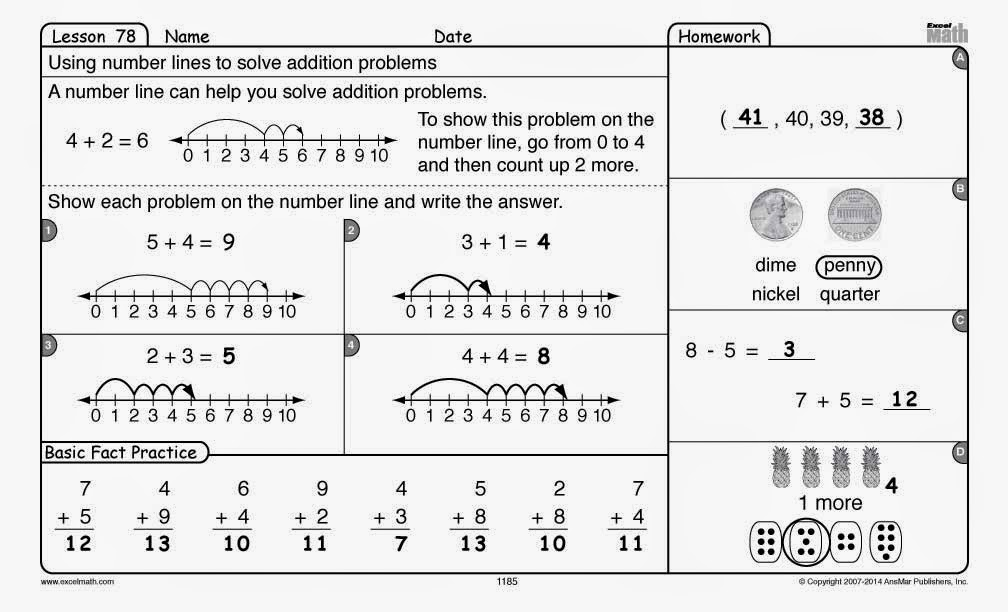## Monday, September 15, 2014

### Back to (Math) Basics

For over 35 years Excel Math has been helping students build solid math skills. And those rigorous math lessons continue to help students succeed today

In Excel Math our Student Lesson Sheets include a section called Basic Fact Practice. Students complete these problems after the Lesson section and before the Guided Practice (unless you choose to use these problems as bell work).Excel Math Student Lesson Sheet with Basic Fact Practice

In this way, basic math facts are practiced regularly. Students have a chance to get basic facts into their long-term memory and continue reviewing them throughout the year.

The Basic Fact Practice portion of the Student Lesson Sheet can be used as a way to start your math period or as bell work so students are on task the minute they enter the classroom. The problems will be fairly easy at first (helping students build confidence) but will increase in difficulty during the year. Excel Math gives students a chance to practice basic facts for several weeks before we ask them to use those facts in complex problems.

Basic Fact Practice is included on the Excel Math Projectable Lesson CDs. The facts can be projected onto a white board or wall so the class can focus together on the problems. The first slide shows the problems and the next slide shows the problems with the answers in red. You can use this section as a daily timed quiz or read the problems aloud before projecting them for aural practice of basic math facts.

Or try our Online Timed Fact Practice:
http://excelmath.com/practice.html

Choose the Excel Math lessons that best fit your students' needs and let us send you a sample:

Common Core Editions—written to guide teachers and students through the new Common Core Standards. Teacher Editions include quarterly test tables that show the CCS concepts covered and in which lesson they were initially taught. See Common Core samples . . .

Texas Editions—a smooth transition to the new TEKS, introducing the new math skills now required at each grade level with our unique spiraling approach to get those concepts into your students' long-term memory. Texas Teacher Editions include quarterly test tables that show the TEKS concepts covered and in which lesson they were initially taught. See Texas samples . . .

Standard Excel Math Editions—our proven lessons with new teacher tips and online resources. Teacher friendly lessons and student successes make it a good fit for any classroom or homeschool situation. See Excel Math Standard Edition samples . . .

You may also enjoy these articles:

Common Core—A New View on Teaching Math

Visit us at the AIE Conference in Austin